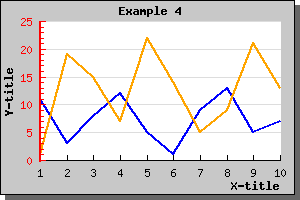Contents Previous Next

### 8.1.3 Adding several plots to the same graph

What if we want to add a second plot to the graph we just produced? Well, this is quite straightforward and just requires two simple step:

1. Create the second plot
2. Add it to the graph

To create the second plot we need some data (we could of course use the same data as for the first plot but then we wouldn't be able to see the new plot!)

The following lines show how to create the new plot and add it to the graph (we only show the new lines - not the full script)

```  \$ydata2  = array(1,19, 15,7,22 ,14,5, 9,21,13 ); \$lineplot2 =new LinePlot(\$ydata2); \$lineplot2 ->SetColor("orange"); \$lineplot2 ->SetWeight(2); \$graph->Add( \$lineplot2); ```

Making these changes to the previous graph script would generate a new graph as illustrated below.Figure 9: Adding a second plot to the previous graph [src]

There is a few things worth noting here

• The Y-scale has changed to accommodate the larger range of Y-values for the second graph.
• If you add several plots to the same graph they should contain the same number of data points. This is not a requirement (the graph will be automatically scaled to accommodate the plot with the largest number of points) but it will not look very good since one of the plot end in the middle of the graph.

Contents Previous Next